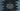# C programming tutorial to learn atof, atoi and atol functions### C programming tutorial to learn atof(), atoi() and atol() functions :

In this C programming tutorial, we will learn three different functions of C : atof(),atoi() and atol(). These functions are mainly used to convert a string to other different data types. Let’s take a look at them with examples :

### atof() :

atof() takes one string as input and it returns one double by converting the input string to double. The syntax of this function is as below :

``double atof(const char* string)``

To use this function, you will have to use stdlib.h header file. Let me show you with one example :

``````#include <stdio.h>
#include <stdlib.h>

int main(){
char number = "12345";

float numberFloat = atof(number);

printf("Floating value is %f ",numberFloat);

}``````

This program will print 12345.000000 as the output .

### atoi() :

atoi() is similar to atof(). It takes one string as the input, convert it to an integer and returns it. The syntax is as below :

``int atoi(const char * str)``

We need to import stdlib for this also.

``````#include <stdio.h>
#include <stdlib.h>

int main(){
char number = "12345";

int numberInt = atoi(number);

printf("Integer value is %d ",numberInt);

}``````

It will print out 12345 as output. If we pass “12.34” as input, it will print 12.

### atol() :

You may have guessed that similar to the above functions, atol() is used to convert a string to a long value. It’s syntax is similar to the above two :

``long int atol(const char * str)``

Let’s modify the above program :

``````#include <stdio.h>
#include <stdlib.h>

int main(){
char number = "12345";

long numberLong = atol(number);

printf("Long value is %ld ",numberLong);

}``````

It will print out 12345 as output. Now if we pass a different string like “abcde”,what it will print ? Let’s take a look :

``````#include <stdio.h>
#include <stdlib.h>

int main(){
char number = "abcde";

float numberFloat = atof(number);
int numberInt = atoi(number);
long numberLong = atol(number);

printf("Float value is %f \n",numberFloat);
printf("Int value is %d \n",numberInt);
printf("Long value is %ld \n",numberLong);

}``````

It will print :

``````Float value is 0.000000
Int value is 0
Long value is 0``````